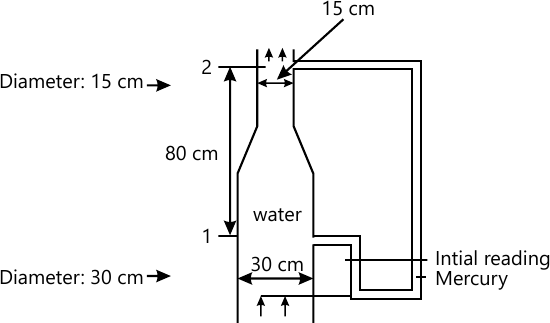VTU Mechanical Engineering (Semester 4)
Fluid Mechanics
June 2012
Total marks: --
Total time: --
INSTRUCTIONS
(1) Assume appropriate data and state your reasons
(2) Marks are given to the right of every question
(3) Draw neat diagrams wherever necessary

1 (a) What is capillarity? derive expression for
capability rise and
capillary depression
6 M
1 (b) What is surface tension? Derive an expression between gauge pressure inside a free jot of liquid and surface tension
6 M
1 (c) Explain bulk modulus of elasticity and vapour pressure
8 M

2 (a) Show that in a fluid rest pressure doe not vary in horizontal direction but various only in vertical direction
6 M
2 (b) Define total pressure and centre of pressure.prove that for an inclined surface submerged in a liquid the centre of pressure always lies centre of gravity
8 M
2 (c) A plane area in the form of right angle triangle of height \'h\'base \'b\'is impressed vertically in water with its vertex at the water surface.calculate the total force on one side of the plane and the location of centre of pressure
6 M

3 (a) With usual notation prove that:
GM=\\frac{I}{\\forall }-BG
10 M
3 (b) determine the condition of stability of a floating body
4 M
3 (c) Determine whether the specified flow is rotational or otherwise ,if irrototional flow determine an expression for the velocity potential function :
u=xy2
6 M

4 (a) State the different forces consider in studying the equation of motion of fluid .State the condition under which each in significant
5 M
4 (b) Derive the Bernaulis equation from
First principle
Euler equation of motion
9 M
4 (c) Water flows up a trapped pipe as shown as Fig .Q4(c) Find the magnitude and direction the deflection 'h' of the differential mercury manometer corresponding to a discharge of 120L.S.P. The friction in the pipe can be completely neglected6 M

5 (a) Derive an expression for discharge over a V-notch
10 M
5 (b) Show by Buckingham theorem the thrust develop by propeller by
$F=\rho V^{2}D^{2}\phi \left [ \frac{\phi D}{V} , Re,M\right ]$
Where =thrust developed ? =angular velocity,V=advance speed ,D=diameter.?viscosity,?=mass density and C=speed of sound.
10 M

6 (a) Differentiate between :
i) Major and minor losses
ii) Hydraulic grade line and total energy line with the help of sketch.
6 M
6 (b) Derive Darcy-Weisbach expression for head loss due to friction in a pipe flow.
8 M
6 (c) A cast iron pipe 20cm in diameter and 600m long connects two reservoirs.if the difference of water levels in the two reservoirs in 30m,find the discharge through the pipe.friction coefficient for the pipe is 0.01.ignore losses other than friction and velocity head.
6 M

7 (a) Starting from first principle derive Hagen-Poisecuille equation for steady laminar flow in a pipe.Further establish relation between Darcy-Weisbach friction factor and Reynolds number for laminar flow
12 M
7 (b) Glycerine (μ=1.5pa-s and ρ=1260kg/m3flows at a velocity of 5m/s in a 10cm diameter pipe .estimate
the boundary shear stress in the pipe due to flow
head loss in a length of 2m length of pipe
power expended by the flow in a distance of 12m
8 M

8 (a) Define the following and give expression for each :
Boundary layer thickness
Displacement thickness
Momentum thickness
Energy thickness
10 M
8 (b) A metallic ball with diameter of 2mm is dropped in liquid of mass density 950kg/m2. Viscosity 15 poise. Specific gravity of ball is 12. Find
drag force on the ball
terminal velocity of the ball.
5 M
8 (c) Determine the velocity of a bullet fired in the atmosphere at 30°C if Match angle is 30°. Take K=1.4,R=287J/kg-K.
5 M

More question papers from Fluid Mechanics